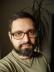## Intersection of Straight Lines

Write a script to find the intersection of two straight lines. The coordinates of the two lines should be provided as command line parameter.

I vaguely remember we did the general form of the equation of a straight line at secondary school. The formula itself is pretty simple:

`Ax + By + C = 0`

Now, clearly, if we have two straight lines `A1, B1, C1` and `A2, B2, C2`, their intersection are the `x` and `y` such that `A1x + B1y + C = A2x + B2y + C2`. From the general formula we know that

`x = (-B1y - C1) / A1`

It takes a bit of pen and paper work to find out that

`y = (A2C1 - C2A1) / (A1B2 - A2B1)`

And we need to pay special attention to the cases where `A1 = 0` or `A1B2 - A2B1 = 0`. In the first case, we’ll use `A2`, `B2`, and `C2` instead to compute `x` (unless `A2 = 0` as well, in which case the two lines are parallel or identical); the second case is similar.

The last part of the puzzle is how to translate the input coordinates of two points into the `A`, `B`, and `C`.

The main trick is to realise that there are infinitely many such triples `A`, `B`, and `C`: we can multiply all of them by any non-zero number to get another triple that still represents the same straight line. We can therefore fix one of the numbers, let’s say `B`, to be always 1 or 0—the latter means the line is vertical. Getting the two remaining numbers is again just a matter of a pen & paper:

```A = (y2 - y1) / (x1 - x2)
C = x1 * (y1 - y2) / (x1 - x2) - y1```

After translating the equations to Perl and adding some tests, we get

``````#!/usr/bin/perl
use warnings;
use strict;
use feature qw{ say };

sub line {
my (\$x1, \$y1, \$x2, \$y2) = @_;
my (\$A, \$B, \$C);
die 'Not enough points' if \$x1 == \$x2 && \$y1 == \$y2;
if (\$x1 == \$x2) {
if (\$x1) {
(\$A, \$B, \$C) = (-1 / \$x1, 0, 1);
} else {
(\$A, \$B, \$C) = (1, 0, 0);
}
} else {
(\$A, \$B, \$C) = ((\$y2 - \$y1) / (\$x1 - \$x2),
1,
\$x1 * (\$y1 - \$y2) / (\$x1 - \$x2) - \$y1);
}
return \$A, \$B, \$C
}

sub intersection {
my (\$a1, \$b1, \$c1, \$a2, \$b2, \$c2) = @_;
if (\$a1 * \$b2 == \$a2 * \$b1) {
die 'No intersection' if \$c1 != \$c2;
die 'Identical lines' if \$c1 == \$c2;
}
my \$y = (\$a2 * \$c1 - \$c2 * \$a1) / (\$a1 * \$b2 - \$a2 * \$b1);
my \$x = \$a1 ? (-\$b1 * \$y - \$c1) / \$a1
: (-\$b2 * \$y - \$c2) / \$a2;
return \$x, \$y
}

use Test::More;
use Test::Exception;

sub point_on_line {
my (\$x1, \$y1, \$x2, \$y2) = @_;
my (\$A, \$B, \$C) = line(\$x1, \$y1, \$x2, \$y2);
is \$A * \$x1 + \$B * \$y1 + \$C, 0;
is \$A * \$x2 + \$B * \$y2 + \$C, 0;
}

point_on_line(3, 3, 5, 3);
point_on_line(3, 3, 3, 7);
point_on_line(1, 5, 3, 11);
throws_ok { line(1, 1, 1, 1) } qr/Not enough points/;

is_deeply [ intersection(
line(1, 5, 3, 11), line(0, -1, 3, 5)
) ], [-3, -7];

is_deeply [ intersection(
line(1, 2, 5, 14), line(0, 2, -1, 7)
) ], [3/8, 1/8];

is_deeply [ intersection(
line(0, 0, 0, 1), line(0, 0, 1, 0)
) ], [0, 0];

is_deeply [ intersection(
line(4.2, 19, 4.8, 22), line(4, 19, 3.5, 17.5)
) ], [4.5, 20.5];

is_deeply [ intersection(
line(1, 1, 5, 1), line(1, 1, 1, 5),
) ], [ 1, 1 ];

throws_ok { intersection(
line(0, 0, 1, 1), line(2, 2, 3, 3)
) } qr/Identical lines/;

throws_ok { intersection(
line(2, 2, 1, 1), line(0, 2, 1, 3)
) } qr/No intersection/;

done_testing(14);``````

This solution doesn’t always work correctly: if the points are very close to each other, the imprecise floating point arithmetic can even claim the points themselves don’t lie on the line. Not even bigrat and similar can fix it if you try something like

``point_on_line(map rand, 1 .. 4) for 1 .. 100;``

## Historical data

Write a script that allows you to capture/display historical data. It could be an object or a scalar. For example
``my \$x = 10; \$x = 20; \$x -= 5;``
After the above operations, it should list the historical values of \$x in order.

In fact, in Perl we can have a scalar that has an object behind itself. To associate a variable with an object, Perl uses the tie function.

To create a scalar that behaves almost like a normal scalar, we can inherit from Tie::StdScalar and only redefine the operations that should behave differently; in our case, we’ll need to modify the STORE method to record the previous value, and the FETCH method to retrieve the last value. The special method TIESCALAR is a constructor of the object, we’ll just bless an empty array into the class.

We can also add new methods to the class—we’ll need one to provide all the historical values. The tied function returns the tied object.

``````#!/usr/bin/perl
use warnings;
use strict;

{   package Historical;
use Tie::Scalar;
use parent -norequire => 'Tie::StdScalar';

sub TIESCALAR { bless [], shift }
sub FETCH     { \$_[-1] }
sub STORE     { push @{ \$_ }, \$_ }

sub history   { \$_ }
}

use Test::More tests => 2;

tie my \$x, 'Historical';
\$x = 'initial value';
\$x = 'second value';
\$x = 'last value';

is_deeply tied(\$x)->history,
[ 'initial value', 'second value', 'last value' ];

tie my \$y, 'Historical';
\$y = 10;
\$y = 20;
\$y -= 5;

is_deeply tied(\$y)->history,
[ 10, 20, 15 ];``````I blog about Perl.